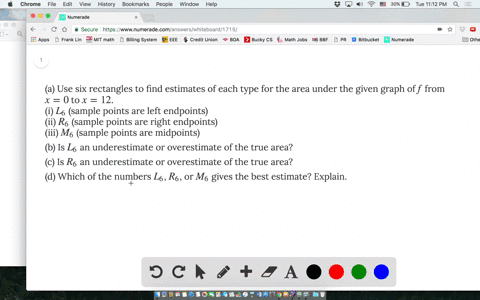### (a) Use six rectangles to find estimates of each …

03:21
FL
Problem 1

(a) By reading values from the given graph of $f$, use five rectangles to find a lower estimate and an upper estimate for the area under the given graph of $f$ from $x = 0$ to $x = 10$. In each case sketch the rectangles that you use.

(b) Find new estimates using ten rectangles in each case.

(A). $R_{5}=11.5$ $L_{5}=21.5$
(B). See explanation for result.

## Discussion

You must be signed in to discuss.

## Video Transcript

So this problem Ask us to estimate the integral off F by using the rectangle message. And if you have the tax, poke it and figure out that function F s a decreasing function. That means for for upper estimate you want to use so that one point because you know the function is decreasing, so the left point will be higher than the right point and similarities. If you want to use a lower estimate, they will have to be the Ryan Point. And once you figure this hours, it's just left to right down the end point off off its track tangos and the pace of its share of tangos. So so for proud, eh? If you want to use our five tangos, there's a chair of tunnel have based, too. And for the upper estimate? No. And it will be, Ah, the base of the structure knows, too a musical by some of height, in this case along to use the left hand points. So it's from fo zero. Remember, Ich Weiss based two's on the next one should be F off, too, and similarly for four I have six half off eight and the lower estimate we'LL have to use the right end point so you appear again faces too and height will be from F off too. And similarly, each one you increase. It's argument by two. So I'll skip the details. The last one should be half of ten and you can figure out the value ofthe the function by looking at the graph on your textbook and so for party. Similarly, the opera estimate it struck tango in this case because used ten off them and the lances ten. I will have the last one and you use the left hand points F zero us if one plus up to F off nine, which is a laugh of m point of the last rectangle and the lower estimate again. The same concept is that we use Ryan Point so it will be a fryer for one. Add up to half of ten